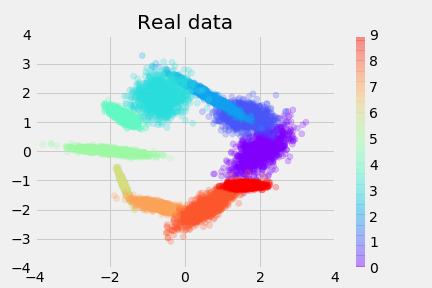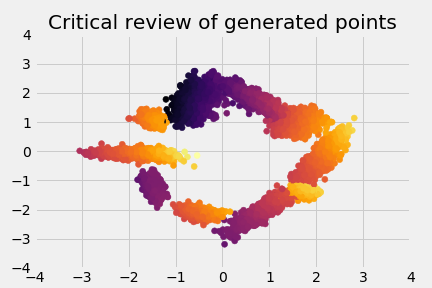I’m trying a new blog format. I’m going to lay these out light science experiments in high school: hypothesis/experiment/results/discussion. We’ll see how it goes.

The notebook for this project can be found here

## Hypothesis

### Introduction

Wassenstein generative adversarial networks minimize the EM-distance between a generated distribution, and a true distribution:

 EM(P(generated data), P(actual data))


A conditional GAN allows one to include a condition (as an input to the generator), that effects the generated data:

 EM(P(generated data|y), P(real data|y))


### Hypothesis

I want to see if I can include the y condition by simply forcing it to be a particular value. The WGAN critic simply the EM distance between the generated data and the real distribution, so by including y in the data, I convert the minimization objective to

 EM(P(generated data, y), P(real data, y))


In effect, I’ve taken away the generator’s ability to control the condition. At the same time, I pass the condition in to the generator, so that it is forced to adapt to the y passed to it. The critic sees the joint distribution of data and condition, so it learns the full joint distribution.

## Method

This is quite easy, and for my experiment I’ve used data from one of 10 2d Gaussians. Here’s the real data-distribution, colored by y, the conditional:The generator’s structure is simply:

Gen (
(layers): ModuleList (
(0): Linear (32 -> 128)
(1): ReLU ()
(2): Linear (128 -> 256)
(3): ReLU ()
(4): Linear (256 -> 256)
(5): ReLU ()
(6): Linear (256 -> 2)
)
)


The critic is:

Critic (
(layers): ModuleList (
(0): Linear (12 -> 256) # 2 inputs from the generator, and 10 one-hot classes.
(1): ReLU ()
(2): Linear (256 -> 256)
(3): ReLU ()
(4): Dropout (p = 0.5)
(5): Linear (256 -> 256)
(6): ReLU ()
(7): Dropout (p = 0.5)
(8): Linear (256 -> 1)
)
)


I use the improved Wasserstien training regularization method, rather than the clipping approach.

During each training step I do the following:

1. Train the critic (x5)
1. Sample a batch from the dataset, of both x and matching ys.
2. Sample from the generator, passing in y
3. Review the sample with the critic, passing in y and the sample.
4. Review the real data with the critic, passing in y and x.
5. Compute the loss, as mean(sample_review) - mean(real_review) + improved wasserstein regularization term.
6. Optimize the critic
2. Sample a batch from the dataset, of both x and matching ys.
3. Sample from the generator, passing in y
4. Review the sample with the critic, passing in y and the sample.
5. Review the real data with the critic, passing in y and x.
6. Compute the loss, as mean(sample_review)
7. Optimize the generator

The notebook for this project can be found here

## Results

This worked great! By the 30-thousandth iteration, the generator was closely matching both the clusters:And the classes of the data:This means I now have a way to feed in conditions to a WGAN, and really easily generate data conditioned on it.

It’s also interesting to look at the scores of the generated points, so these are the “reviews” of the data:## Discussion

This leaves a few open questions:

1. Is it possible to double-down and make a generative process for ys. In this case we drew samples of y from the dataset, but perhaps it would be better to generate both data and y values, but generate y values in a separate module so that later I can set conditions.
2. Does it work for MNIST? And CIFAR?
3. Is it possible to condition on continuous values? This is easy to test as a complex multiple regression problem.

## Conclusion

It’s quite easy to make a conditional-WGAN, by simply:

1. Passing the conditions to the data-generator.
2. Also passing the conditions (and the generated data) to the critic when scoring fake data.
3. Passing the real-data and matching conditions to the critic when scoring real data.